# How To Convert Infinity, -Infinity, NaN to Zero ValueSometimes in the numeric formatted cells, as a result of various calculations, it is possible to have returned as a result the Anaplan special numbers: Infinity, -Infinity, or NaN (Not a Number).

If the numeric line item with this issue is also an input for other formulas, the final result could be altered. To solve this issue a solution is to convert these Anaplan special numbers in zero values.

## Convert Infinity and -Infinity:

First thing is to have calculated the "Infinity" value in a system module without any lists.

This can be achieved in different ways by applying one of the formulas in a numeric formatted line-item: DIVIDE(1,0) or VALUE("Infinity").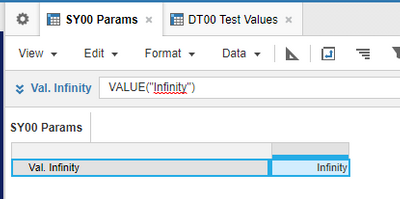Then it can be applied, in sequence, to the following Anaplan functions:

1. Convert numeric line-item in positive number  (function: ABS).
2. Compare the result in Step 1 if it is NOT equal with the Infinity value calculated in the system module
3. If TRUE then consider the numeric value, ELSE consider 0

Example: IF ABS(Value num) <> 'SY00 Params'.'Val. Infinity' THEN Value num ELSE 0

Note: ABS function is useful to solve in one comparison both Infinity (positive) and -Infinity (negative)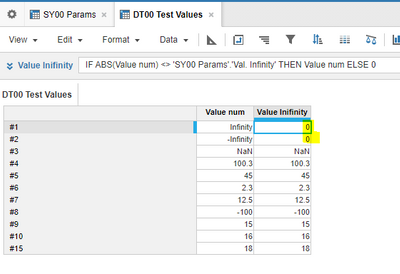Convert NaN: Apply in sequence the following Anaplan functions/statements:

1. Convert numeric line-item in positive number  (function: ABS)
2. Compare result Step 1 if it is greater or equal with zero. NaN value will return false.
3. Consider the initial value of the line-item if Step 1 is greater or equal with zero else consider zero (on ELSE the NaN will be converted to zero).

Example: IF ABS(Value num) >= 0 THEN Value num ELSE 0

Notes:

- ABS function is useful to solve in one comparison both positive and negative numbers

- NaN=NaN, NaN>=0, NaN<=0 comparisons will always return FALSE. This is why it is needed to check with zero the numeric values.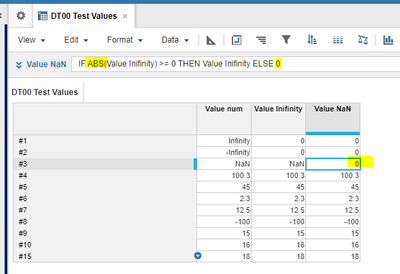Tagged:

•Thanks for sharing. This is a great hack / help 🙂

AB

•Thanks for this. I have seen this problem in one particular module in one particular model. I will see about implementing this.

•Hi @alexpavel,

I like your solution if you want to exclude NaNs OR infinities. However, if you want to zero both NaNs AND infinities, I prefer less complicated (just one simple IF, no SYS modules) solution:

IF Value * 0 = 0 THEN Value ELSE 0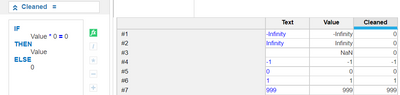Here I utilize properties of multiplication by 0. "Normal" numbers give 0, but NaN and infinities times 0 are NaN.

•I like your solution too..Indeed multiplying Infinity, -Infinity with zero will return NaN...and then comparing with zero arrive at the same result 🙂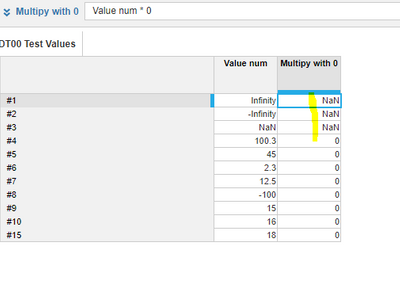•Nice hack.  Will use this in future.

•One callout. If you want to reference "Infinity" in the formula just remember to have a clause for "-Infinity" as well. that way both will convert to 0 or whatever you need.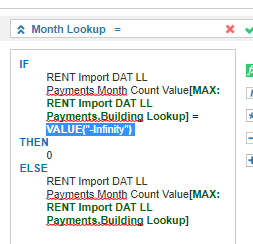•@M.Kierepka - great hack!

I had about a million NaN and Infinity formulas running through a number of modules due to a recovery formula trying to calculate without throughput (a few years in the future) - I just had to apply your trick formula to one line item in one module and it corrected the calculations throughout the model. Thanks for the tip!